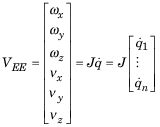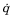# geometricJacobian

Geometric Jacobian for robot configuration

## Syntax

``jacobian = geometricJacobian(robot,configuration,endeffectorname)``

## Description

example

````jacobian = geometricJacobian(robot,configuration,endeffectorname)` computes the geometric Jacobian relative to the base for the specified end-effector name and configuration for the robot model.```

## Examples

collapse all

Calculate the geometric Jacobian for a specific end effector and configuration of a robot.

Load a PUMA 560 robot from the Robotics System Toolbox™ `loadrobot`, specified as a `rigidBodyTree` object.

`puma = loadrobot("puma560");`

Calculate the geometric Jacobian of body "`link7"` on the Puma robot for a random configuration.

`geoJacob = geometricJacobian(puma,randomConfiguration(puma),"link7")`
```geoJacob = 6×6 -0.0000 -0.5752 -0.5752 -0.4266 -0.7683 -0.5213 0.0000 0.8180 0.8180 -0.3000 -0.3776 0.8377 1.0000 -0.0000 -0.0000 0.8533 -0.5168 0.1629 0.1696 0.0823 0.3087 -0.0407 0.0198 0 -0.5577 0.0578 0.2171 -0.0200 0.0210 0 0.0000 0.5538 0.2224 -0.0274 -0.0448 0 ```

## Input Arguments

collapse all

Robot model, specified as a `rigidBodyTree` object.

Robot configuration, specified as a vector of joint positions or a structure with joint names and positions for all the bodies in the robot model. You can generate a configuration using `homeConfiguration(robot)`, `randomConfiguration(robot)`, or by specifying your own joint positions in a structure. To use the vector form of `configuration`, set the `DataFormat` property for `robot` to either `"row"` or `"column"`.

End-effector name, specified as a string scalar or character vector. An end effector can be any body in the robot model.

Data Types: `char` | `string`

## Output Arguments

collapse all

Geometric Jacobian of the end effector with the specified `configuration`, returned as a 6-by-n matrix, where n is the number of degrees of freedom of the robot. The Jacobian maps the joint-space velocity to the end-effector velocity, relative to the base coordinate frame. The end-effector velocity equals:

``

ω is the angular velocity, υ is the linear velocity, andis the joint-space velocity.

collapse all

### Dynamics Properties

When working with robot dynamics, specify the information for individual bodies of your manipulator robot using these properties of the `rigidBody` objects:

• `Mass` — Mass of the rigid body in kilograms.

• `CenterOfMass` — Center of mass position of the rigid body, specified as a vector of the form `[x y z]`. The vector describes the location of the center of mass of the rigid body, relative to the body frame, in meters. The `centerOfMass` object function uses these rigid body property values when computing the center of mass of a robot.

• `Inertia` — Inertia of the rigid body, specified as a vector of the form `[Ixx Iyy Izz Iyz Ixz Ixy]`. The vector is relative to the body frame in kilogram square meters. The inertia tensor is a positive definite matrix of the form:The first three elements of the `Inertia` vector are the moment of inertia, which are the diagonal elements of the inertia tensor. The last three elements are the product of inertia, which are the off-diagonal elements of the inertia tensor.

For information related to the entire manipulator robot model, specify these `rigidBodyTree` object properties:

• `Gravity` — Gravitational acceleration experienced by the robot, specified as an `[x y z]` vector in m/s2. By default, there is no gravitational acceleration.

• `DataFormat` — The input and output data format for the kinematics and dynamics functions, specified as `"struct"`, `"row"`, or `"column"`.

### Dynamics Equations

Manipulator rigid body dynamics are governed by this equation:

`$\frac{d}{dt}\left[\begin{array}{c}q\\ \stackrel{˙}{q}\end{array}\right]=\left[\begin{array}{c}\stackrel{˙}{q}\\ M{\left(q\right)}^{-1}\left(-C\left(q,\stackrel{˙}{q}\right)\stackrel{˙}{q}-G\left(q\right)-J{\left(q\right)}^{T}{F}_{Ext}+\tau \right)\end{array}\right]$`

also written as:

`$M\left(q\right)\stackrel{¨}{q}=-C\left(q,\stackrel{˙}{q}\right)\stackrel{˙}{q}-G\left(q\right)-J{\left(q\right)}^{T}{F}_{Ext}+\tau$`

where:

• $M\left(q\right)$ — is a joint-space mass matrix based on the current robot configuration. Calculate this matrix by using the `massMatrix` object function.

• $C\left(q,\stackrel{˙}{q}\right)$ — are the Coriolis terms, which are multiplied by $\stackrel{˙}{q}$ to calculate the velocity product. Calculate the velocity product by using by the `velocityProduct` object function.

• $G\left(q\right)$ — is the gravity torques and forces required for all joints to maintain their positions in the specified gravity `Gravity`. Calculate the gravity torque by using the `gravityTorque` object function.

• $J\left(q\right)$ — is the geometric Jacobian for the specified joint configuration. Calculate the geometric Jacobian by using the `geometricJacobian` object function.

• ${F}_{Ext}$ — is a matrix of the external forces applied to the rigid body. Generate external forces by using the `externalForce` object function.

• $\tau$ — are the joint torques and forces applied directly as a vector to each joint.

• $q,\stackrel{˙}{q},\stackrel{¨}{q}$ — are the joint configuration, joint velocities, and joint accelerations, respectively, as individual vectors. For revolute joints, specify values in radians, rad/s, and rad/s2, respectively. For prismatic joints, specify in meters, m/s, and m/s2.

To compute the dynamics directly, use the `forwardDynamics` object function. The function calculates the joint accelerations for the specified combinations of the above inputs.

To achieve a certain set of motions, use the `inverseDynamics` object function. The function calculates the joint torques required to achieve the specified configuration, velocities, accelerations, and external forces.

 Featherstone, Roy. Rigid Body Dynamics Algorithms. Springer US, 2008. DOI.org (Crossref), doi:10.1007/978-1-4899-7560-7.